Test: Frequency Filters

# Test: Frequency Filters

Test Description

## 10 Questions MCQ Test GATE ECE (Electronics) 2023 Mock Test Series | Test: Frequency Filters

Test: Frequency Filters for Electronics and Communication Engineering (ECE) 2023 is part of GATE ECE (Electronics) 2023 Mock Test Series preparation. The Test: Frequency Filters questions and answers have been prepared according to the Electronics and Communication Engineering (ECE) exam syllabus.The Test: Frequency Filters MCQs are made for Electronics and Communication Engineering (ECE) 2023 Exam. Find important definitions, questions, notes, meanings, examples, exercises, MCQs and online tests for Test: Frequency Filters below.
Solutions of Test: Frequency Filters questions in English are available as part of our GATE ECE (Electronics) 2023 Mock Test Series for Electronics and Communication Engineering (ECE) & Test: Frequency Filters solutions in Hindi for GATE ECE (Electronics) 2023 Mock Test Series course. Download more important topics, notes, lectures and mock test series for Electronics and Communication Engineering (ECE) Exam by signing up for free. Attempt Test: Frequency Filters | 10 questions in 30 minutes | Mock test for Electronics and Communication Engineering (ECE) preparation | Free important questions MCQ to study GATE ECE (Electronics) 2023 Mock Test Series for Electronics and Communication Engineering (ECE) Exam | Download free PDF with solutions
 1 Crore+ students have signed up on EduRev. Have you?
Test: Frequency Filters - Question 1

### Consider the circuit below and find its cut-off frequency.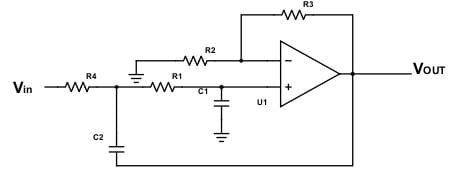R1=10kΩ, R2=20kΩ, R3=30kΩ, C1=2nF, C2=4nF, R4=15kΩ.

Detailed Solution for Test: Frequency Filters - Question 1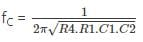fC = 4.6 kHz.

Test: Frequency Filters - Question 2

### For a low pass filter of non-inverting type, the cutoff frequency is 2kHz and the input frequency is 4kHz. Find the phase shift in output.

Detailed Solution for Test: Frequency Filters - Question 2

Phase shift = -tan-1f/fC = -tan-12 = -63°.

Test: Frequency Filters - Question 3

### What is the frequency response of the filter shown below?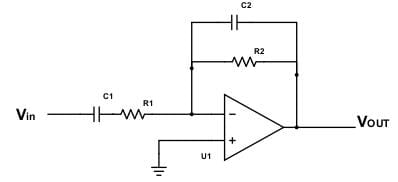Detailed Solution for Test: Frequency Filters - Question 3

The given circuit is a bandpass filter. The upper and lower cut-off frequencies depend on the RC constant. However, since R1C1 = R2C2, this means that both the cut-off frequencies are equal. Thus they meet at the same point and form the response as above.

Test: Frequency Filters - Question 4

When the input frequency is equal to the cutoff frequency, how much is the phase shift in the output?

Detailed Solution for Test: Frequency Filters - Question 4

Phase shift = 180° – tan-1f/fC
Phase shift = 180-45 = 135°.

Test: Frequency Filters - Question 5

In a low pass filter as below, find the cut-off frequency for the following circuit.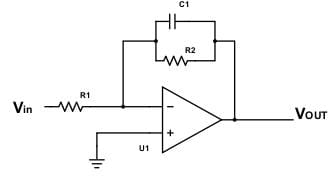Given that R1=20kΩ, R2=25kΩ, C1=10nF.

Detailed Solution for Test: Frequency Filters - Question 5

The cut-off frequency for the above filter is fc = 1/2πR2C1
fC = 1000000/2π25×10 = 636 Hz.

Test: Frequency Filters - Question 6

A filter is provided of order 3, find the roll-off rate.

Detailed Solution for Test: Frequency Filters - Question 6

Roll-off rate is the rate at which the gain of a filter decreases outside the pass-band. Roll of rate is increased as the order increases. For an nth order filter, roll-off rate is 20xn dB/decade.

Test: Frequency Filters - Question 7

Given the following circuit, find the maximum gain.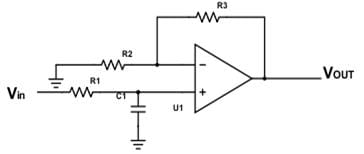Detailed Solution for Test: Frequency Filters - Question 7

The above circuit is a low pass filter of the non-inverting type, where the input is at the non-inverting end. The gain is A = 1+R3/R2 / 1+jωR1C1
Thus maximum gain is A = 1+R3/R2.

Test: Frequency Filters - Question 8

For the circuit, calculate the phase shift.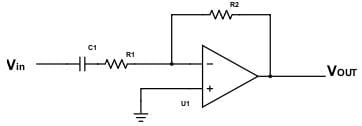It is given that R1=20kΩ, C1=2nF, R2=22kΩ, and input signal is 2sin6π103t.

Detailed Solution for Test: Frequency Filters - Question 8

Net phase shift is 180 + tan-1fC/f
fc = 1/2πR1C1 = 1000000/2π20×2 = 3.978 kHz
Phase shift = 180 + tan-13.987/3 = 233.04°.

Test: Frequency Filters - Question 9

Given that the maximum gain of a low pass filter using op-amp is 5.5 and the resistor R1 = 10kΩ, find the value of R2.

Detailed Solution for Test: Frequency Filters - Question 9

The maximum gain is at low frequencies and at low frequencies, the capacitance reactance is infinite and thus the total feedback impedance ZF = RF = R2
Thus gain = -ZF/Z = -R2/R1
Amplitude = 5.5 = R2/R1 = R2/10k
R2 = 55kΩ.

Test: Frequency Filters - Question 10

Which is not a difference between active and passive filter?

Detailed Solution for Test: Frequency Filters - Question 10

A passive filter can consist of all R, L and C elements. An op-amp is used in an active filter, and it also provides amplification along with filtering. There are no inductors used in active filters because they are bigger in size and bulky. Active filters are used at audio frequency and passive filter at radio frequency.

## GATE ECE (Electronics) 2023 Mock Test Series

20 docs|261 tests
 Use Code STAYHOME200 and get INR 200 additional OFF Use Coupon Code
Information about Test: Frequency Filters Page
In this test you can find the Exam questions for Test: Frequency Filters solved & explained in the simplest way possible. Besides giving Questions and answers for Test: Frequency Filters, EduRev gives you an ample number of Online tests for practice

## GATE ECE (Electronics) 2023 Mock Test Series

20 docs|261 tests